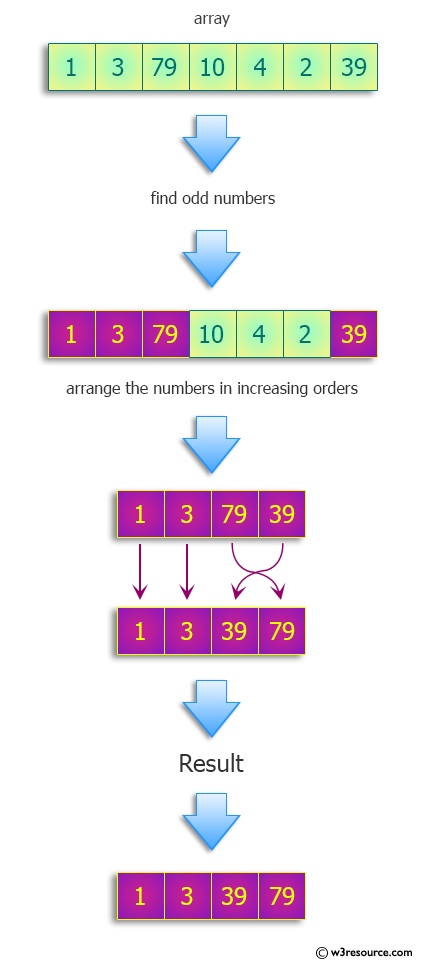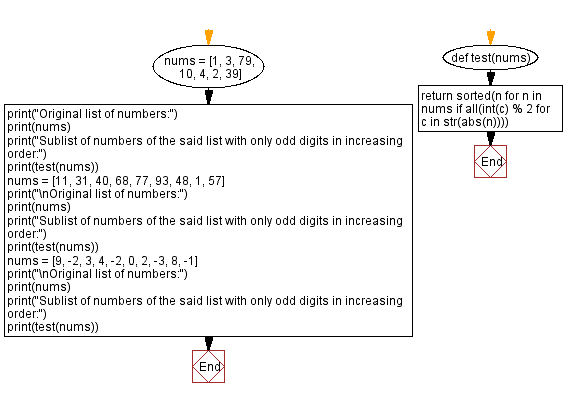﻿ Python: Find the sublist of numbers with only odd digits in increasing order - w3resource# Python: Find the sublist of numbers with only odd digits in increasing order

## Python Programming Puzzles: Exercise-82 with Solution

Write a Python program to find the sublist of numbers from a given list of numbers with only odd digits in increasing order.

```Input:
[1, 3, 79, 10, 4, 2, 39]
Output:
[1, 3, 39, 79]

Input:
[11, 31, 40, 68, 77, 93, 48, 1, 57]
Output:
[1, 11, 31, 57, 77, 93]

Input:
[9, -2, 3, 4, -2, 0, 2, -3, 8, -1]
Output:
[-3, -1, 3, 9]
```

Pictorial Presentation:Sample Solution:

Python Code:

``````#License: https://bit.ly/3oLErEI

def test(nums):
return sorted(n for n in nums if all(int(c) % 2 for c in str(abs(n))))

nums = [1, 3, 79, 10, 4, 2, 39]
print("Original list of numbers:")
print(nums)
print("Sublist of numbers of the said list with only odd digits in increasing order:")
print(test(nums))
nums = [11, 31, 40, 68, 77, 93, 48, 1, 57]
print("\nOriginal list of numbers:")
print(nums)
print("Sublist of numbers of the said list with only odd digits in increasing order:")
print(test(nums))
nums = [9, -2, 3, 4, -2, 0, 2, -3, 8, -1]
print("\nOriginal list of numbers:")
print(nums)
print("Sublist of numbers of the said list with only odd digits in increasing order:")
print(test(nums))
``````

Sample Output:

```Original list of numbers:
[1, 3, 79, 10, 4, 2, 39]
Sublist of numbers of the said list with only odd digits in increasing order:
[1, 3, 39, 79]

Original list of numbers:
[11, 31, 40, 68, 77, 93, 48, 1, 57]
Sublist of numbers of the said list with only odd digits in increasing order:
[1, 11, 31, 57, 77, 93]

Original list of numbers:
[9, -2, 3, 4, -2, 0, 2, -3, 8, -1]
Sublist of numbers of the said list with only odd digits in increasing order:
[-3, -1, 3, 9]
```

Flowchart:## Visualize Python code execution:

The following tool visualize what the computer is doing step-by-step as it executes the said program:

Python Code Editor :

Have another way to solve this solution? Contribute your code (and comments) through Disqus.

What is the difficulty level of this exercise?

Test your Programming skills with w3resource's quiz.

﻿

## Python: Tips of the Day

Clamps num within the inclusive range specified by the boundary values x and y:

Example:

```def tips_clamp_num(num,x,y):
return max(min(num, max(x, y)), min(x, y))
print(tips_clamp_num(2, 4, 6))
print(tips_clamp_num(1, -1, -6))
```

Output:

```4
-1
```